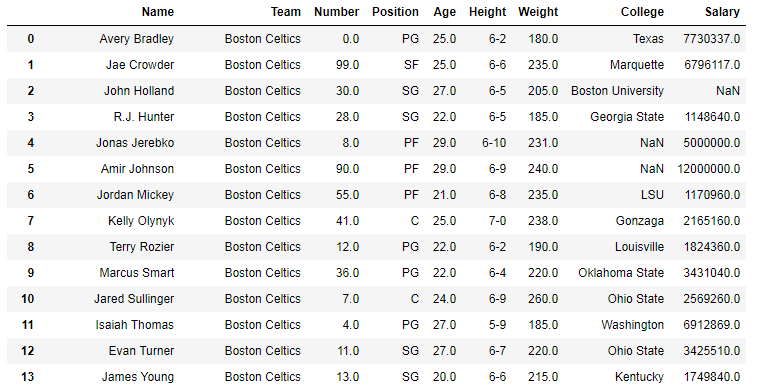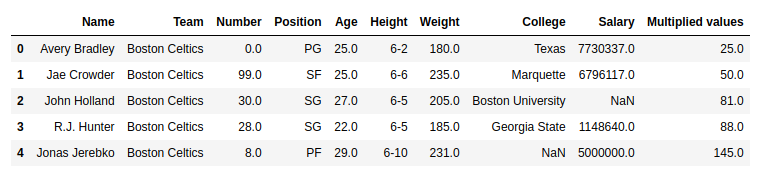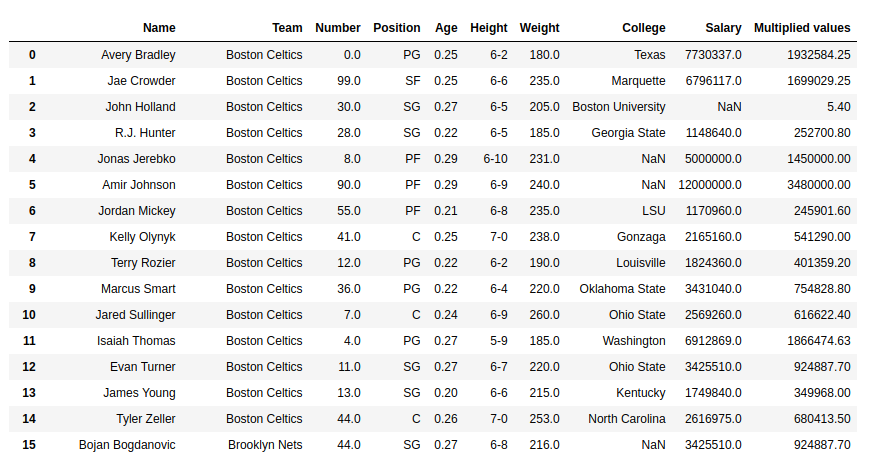Skip to content
Related Articles
Python | Pandas Series.mul()
• Last Updated : 01 Oct, 2018

Python is a great language for doing data analysis, primarily because of the fantastic ecosystem of data-centric Python packages. Pandas is one of those packages and makes importing and analyzing data much easier.

Python `Series.mul()` is used to multiply series or list like objects with same length with the caller series.

Syntax: Series.mul(other, level=None, fill_value=None, axis=0)

Parameters:
other: other series or list type to be multiplied with caller series
fill_value: Value to be replaced by NaN in series/list before multiplication
level: integer value of level in case of multi index

Return type: Caller series with multiplied values

To download the data set used in following example, click here.
In the following examples, the data frame used contains data of some NBA players. The image of data frame before any operations is attached below.Example #1: Multiplying list with series

In this example, the top 5 rows are stored in new variable using .head() method. After that a list of same length is created and multiplied with the Age column using .mul() method

 `# importing pandas module ``import` `pandas as pd`` ` `# reading csv file from url ``data ``=` `pd.read_csv(``"https://media.geeksforgeeks.org/wp-content/uploads/nba.csv"``)`` ` `# creating short data of 5 rows``short_data ``=` `data.head()`` ` `# creating list with 5 values``list` `=``[``1``, ``2``, ``3``, ``4``, ``5``]`` ` `# multiplying list data``# creating new column``short_data[``"Multiplied values"``]``=` `short_data[``"Age"``].mul(``list``)`` ` `# display``short_data`

Output:
As shown in the output image, it can be compared that the Multiplied values column is having the Multiplied values of (Age) x (list).Example #2: Multiplying series with series having null values

In this example, the Salary column is multiplied with the Age column. Since the values in both Salary and Age column are large, product will be returned with high value. Hence just for demonstrating purposes, the age column is divided with 100 before doing the multiplication. Since the salary column contains null values too, by default it returns NaN no matter what is multiplied. In this example, 20 is passed to replace null values with 20.

 `# importing pandas module ``import` `pandas as pd`` ` `# reading csv file from url ``data ``=` `pd.read_csv(``"https://media.geeksforgeeks.org/wp-content/uploads/nba.csv"``)`` ` `# dividing age series``data[``"Age"``]``=` `data[``"Age"``]``/``100`` ` `age ``=` `data[``"Age"``]`` ` `# na replacement``na ``=` `20`` ` `# Multiplying values``# storing to new column``data[``"Multiplied values"``]``=` `data[``"Salary"``].mul(other ``=` `age, fill_value ``=` `na)`` ` `# display``data`

Output:
As shown in the output image, the Multiplicated value column has multiplied age column with 20 in case of Null values.Attention geek! Strengthen your foundations with the Python Programming Foundation Course and learn the basics.

To begin with, your interview preparations Enhance your Data Structures concepts with the Python DS Course. And to begin with your Machine Learning Journey, join the Machine Learning – Basic Level Course

My Personal Notes arrow_drop_up